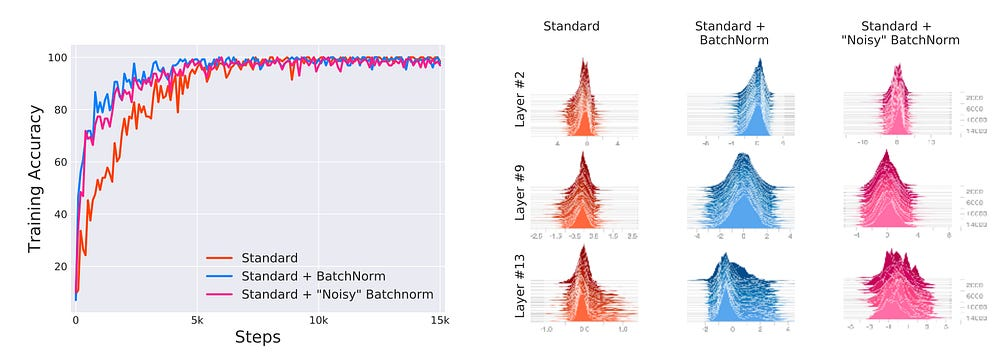A new understanding of Batch Normalization

Batch Normalization(BN) was introduced by  back in 2015. Since then it has been used in the most deep learning models to improve training and robustness to the selection of learning rate as well as parameter initialization.

BN was designed to reduce Internal Covariate Shift (ICS) of each layer ’s input by normalizing the first two moments — mean and variance. At the same time not affecting the network’s ability to produce the desired distribution of activation by using a pair of learnable parameters (gamma and beta).

A recent paper , sheds some new light on BN and performance gain obtained by using the normalization technique. Based on experiments, it reports:

• ICS is not a good predictor of training performance
• Performance gain obtained using BN does not stem from a reduction in ICS
• BN rather provides a smoothing effect on the optimization landscape, which improves models robustness to hyperparameter such as learning rate.

Experiment 1

Figure 1 below (taken from ) shows three sets of training a VGG network. The first network is trained without BN, second trained with BN; lastly, the third network is injected with distributional instability after each BN used by adding time-varying, non-zero mean and non-unit variance noise. The noise essentially causes a high ICS, possibly higher than the standard setting.

The results demonstrate that even with increased ICS by addition of noise, the performance gain is still obtained (pink line). This points to reduction in ICS not being the factor causing improvement in performance.

Experiment 2

For each neural network layer, ICS captures the change in optimization problem itself caused due to change in inputs to each layer as the parameters of previous layers are updated using gradient descent. As a reaction of this ‘shift’, each layer needs to adjust its parameters, often causing vanishing or explosion of gradients .

This idea of change in optimization landscape would also be reflected by changes in gradients of the layer’s parameters. Higher change in gradient would reflect a bigger change in optimization landscape.  captures this by measuring the difference between the gradients of each layer before (G) and after updates to all the previous layers (G’). A smaller value of the l2 difference would indicate a smaller ICS, as the landscape remains similar.

 further investigates link between ICS and BN by plotting l2 difference (and cosine angle) of the two gradients, seen in figure 2. From the figure above it can be seen that using BN does not indicate a reduction in ICS.

So what does Batch Normalization do then?

An Deep Neural Network’s optimization landscape may consist of numerous flat regions and sharp kinks, which make the problem non-convex. Such regions lead to vanishing gradient (flat regions) or gradient explosion (sharp slopes). This increases sensitivity to the learning rate and initialization of parameters, making the optimization unstable.

 refers to higher Lipschitzness of the gradients using BN, which effectively means a higher smoothness of the optimization landscape. This can be observed in figure 3, which plots computes the gradient of the loss at a training step and measures how the loss changes along that gradient direction.